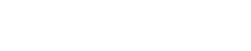# Factorial: Simple Definition, Examples & Distribution

Contents:

## What is a Factorial?

Watch the video for a definition and how to calculate factorials.

Watch this video on YouTube.

Factorials (!) are products of every whole number from 1 to n. In other words, take the number and multiply through to 1.

For example:

• If n is 3, then 3! is 3 x 2 x 1 = 6.
• If n is 5, then 5! is 5 x 4 x 3 x 2 x 1 = 120.

It’s a shorthand way of writing numbers. For example, instead of writing 479001600, you could write 12! instead (which is 12 x 11 x 10 x 9 x 8 x 7 x 6 x 5 x 4 x 3 x 2 x 1).

### What is a factorial used for in stats?

In algebra, you probably encountered ugly-looking factorials like (x – 10!)/(x + 9!). Don’t worry; You won’t be seeing any of these in your beginning stats class. Phew! The only time you’ll see them is for permutation and combination problems.

Related article (from CalculusHowTo.com): Why does Zero Factorial Equal One?

## Factorial Distribution

A factorial distribution happens when a set of variables are independent events. In other words, the variables don’t interact at all; Given two events x and y, the probability of x doesn’t change when you factor in y. Therefore, the probability of x, given that y has happened —P(x|y)— will be the same as P(x).

The factorial distribution can be written in many ways (Hinton, 2013; Olshausen, 2004):

• p(x,y) = p(x)p(y)
• p(x,y,z) = p(x)p(y)p(z)
• p(x1, x2, x3, x4) = p(x1) P(x2) p(x3) p(x4)

In the case of a probability vector, the meaning is exactly the same. That is, a probability vector from a factorial distribution is the product of probabilities of the vector’s individual terms.

You may notice a lack of the factorial symbol (!) in any of the definitions. That’s because the distribution is named because successive frequencies are factorial quantities, rather than the terms being factorials themselves.

## Defining a Factorial Distribution

For a factorial distribution, P(x,y) = P(x)P(y). We can generalize this for more than two variables (Olshausen, 2004) and write:

P(x1, x2,…,xn) = P(x1) · P(x2 · …· P(xn).

This expression can also be written more concisely as:

P(x1, x2,…xn)= ΠiP(xi).

## Factorial Distribution Examples

We like to work with factorial distributions because their statistics are easy to compute. In some fields such as neurology, situations best represented by complicated, intractable probability distributions are approximated by factorial distributions in order to take advantage of this ease of manipulation.

One example of an often-encountered factorial distribution is the p-generalized normal distribution, represented by the equation

I won’t go into the meaning of that formula here; if you’d like to go deeper, feel free to read up on it here. But note that when p = 2, this is exactly the normal distribution. So the normal distribution is also factorial.

## Gamma Function

The Gamma function (sometimes called the Euler Gamma function) is related to factorials by the following formula:

Γ(n) = (x – 1)!.

In other words, the gamma function is equal to the factorial function. However, while the factorial function is only defined for non-negative integers, the gamma can handle fractions as well as complex numbers.

The multivariate gamma function (MGF) is an extension of the gamma function for multiple variables. While the gamma function can only handle one input (“x”), the multivariate version can handle many. It is usually defined as:## References

Abramowitz, M. and Stegun, I. A. (Eds.). “Gamma (Factorial) Function” and “Incomplete Gamma Function.” §6.1 and 6.5 in Handbook of Mathematical Functions with Formulas, Graphs, and Mathematical Tables, 9th printing. New York: Dover, pp. 255-258 and 260-263, 1972.
Grötschel, M. et al. (Eds.) (2013). Online Optimization of Large Scale Systems. Springer Science & Business Media.
Hinton, G. (2013). Lecture 1: Introduction to Machine Learning and Graphical Models. Retrieved December 28, 2017 from: https://www.cs.toronto.edu/~hinton/csc2535/notes/lec1new.pdf
Jordan, I. et al. (2001). Graphical Models: Foundations of Neural Computation. MIT Press.
Olshausen, B. (2004). A Probability Primer. Retrieved December 27, 2017 from: